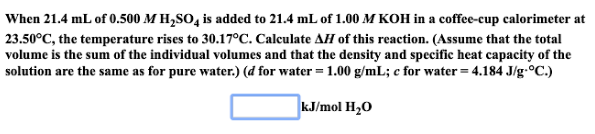# When 21.4 mL of 0.500 M H2SO4 is added to 21.4 mL of 1.00 M KOH in ah coffee-cup calorimeter at 23.50 degrees C, the temperature rises to 30.17 degrees C. Calculate ΔH of this reaction. (Assume that the total volume is the sum of the individual volumes and that the density and specific heat capacity of the solution are the same as for pure water.) (d for water = 1.00 g/mL; c for water = 4.184 J/g degrees C.)